## Repeat an Algorithm Using a For-Each Subsystem

To repeat an algorithm, you can iterate the algorithm over signals, subsystems, and parameters that are grouped into arrays and structures. This page gives examples of how to convert an inefficiently complex repetitive algorithm into a compact form that is easier to manage.

If you repeat algorithms in a diagram by copying and pasting blocks and subsystems, maintaining the model can become difficult. Individual signal lines and subsystems can crowd the diagram, reducing readability and making simple changes difficult. Variables can also crowd workspaces, reducing model portability. A model can develop these efficiency issues as you add to the design over time.

### Explore Example Model

The model `ex_repeat_algorithm` processes and analyzes sensor data from three burners containing three sensors each, using the same algorithms for each burner in separate, identical subsystems. The model can be simplified by using for-each subsystems.

1. Open the example model `ex_repeat_algorithm`. The model creates about 30 variables in the base workspace.

2. Inspect the subsystem named `Burner_1_Analysis`. This subsystem executes an algorithm by using the base workspace variables as parameters in blocks such as Constant and Discrete-Time Integrator blocks.

3. Inspect the subsystems named `Burner_2_Analysis` and `Burner_3_Analysis`. All three subsystems execute the same algorithm but use different workspace variables to parameterize the blocks.

4. Inspect the three `Analysis_Delay` subsystems. These subsystems repeat a different algorithm from the one in the `Analysis` subsystems.

5. Return to the top level of the model. The Memory blocks delay the input signals before they enter the `Analysis_Delay` subsystems.

6. Look at the Data Import/Export pane of the Configuration Parameters dialog box. The model uses the variables `SensorsInput` and `t` as simulation inputs.

During simulation, each of the nine columns in the matrix variable `SensorsInput` provides input data for an Inport block at the top level of the model.

### Reduce Signal Line Density with Buses

You can use buses to group related signals into a single structured signal, reducing line density and improving model readability.

Each subsystem in the example model requires three signal inputs. You can combine each group of three signals into a single bus.

You can modify all the subsystems in the example model to use buses. However, because some of the subsystems are identical, you can delete them and later replace them with For Each Subsystem blocks.

1. Open the Type Editor.

`typeeditor`

2. Create a `Simulink.Bus` object in the base workspace named `SensorData` with three elements: `sensor1`, `sensor2`, and `sensor3`.3. Delete the blocks as shown in the figure. Leave only the blocks named `Burner_1_Sensor1` and `Burner_1_Delay1` as inputs to the two remaining subsystems.4. On the Signal Attributes tab of the `Burner_1_Sensor1` Inport block dialog box, set Data type to `Bus: SensorData`.

The output of the block is a bus that contains the three signal elements `sensor1`, `sensor2`, and `sensor3`.

5. Open the subsystem named `Burner_1_Analysis`. Delete the signal output lines of the three Inport blocks. Delete the Inport blocks named `In2` and `In3`.

6. Add a Bus Selector block to the right of the Inport block named `In1`. Connect the Inport block output to the Bus Selector block.

7. In the Bus Selector block dialog box, select the signals `sensor1`, `sensor2`, and `sensor3`.The Bus Selector block extracts the three signal elements from the input bus. Other blocks in the model can use the extracted signal elements.

8. In the subsystem, connect the blocks as shown.9. In the subsystem named `Burner_1_Analysis_Delay`, use a Bus Selector block to extract the signals in the bus. Use the same technique as you did in the subsystem named `Burner_1_Analysis`.

### Repeat an Algorithm

A For Each Subsystem block partitions an input signal and sequentially executes an algorithm on each partition. For example, if the input to the subsystem is an array of six signals, you can configure the subsystem to execute the same algorithm on each of the six signals.

You can use for-each subsystems to repeat an algorithm in an iterative fashion. This approach improves model readability and makes it easy to change the repeated algorithm .

1. Add two For Each Subsystem blocks to the model. Name one of the subsystems `Burner_Analysis`. Name the other subsystem `Burner_Analysis_Delay`.

2. Copy the contents of the subsystem named `Burner_1_Analysis` into the subsystem named `Burner_Analysis`. Before you paste the blocks, delete the Inport and Outport blocks in the for-each subsystem.

3. In the For Each block dialog box in the `Burner_Analysis` subsystem, select the check box to partition the input `In1`.

4. Copy the contents of the subsystem named `Burner_1_Analysis_Delay` into the subsystem named `Burner_Analysis_Delay`.

5. In the For Each block dialog box in the `Burner_Analysis_Delay` subsystem, select the check box to partition the input `In1`.

6. At the top level of the model, delete the subsystems named `Burner_1_Analysis` and `Burner_1_Analysis_Delay`. Connect the new For Each Subsystem blocks in their place.

7. On the Signal Attributes tab of the `Burner_1_Sensor1` Inport block dialog box, set Port dimensions to `3`.

The block output is a three-element array of buses. The for-each subsystems in the model repeat an algorithm for each of the three buses in the array.

8. Create a `Simulink.SimulationData.Dataset` object that the Inport block can use to import the simulation data. You can use this code to create the object and store it in the variable `SensorsInput`.

```% First, create an array of structures whose field values are % timeseries objects. for i = 1:3 % Burner number % Sensor 1 eval(['tempInput(1,' num2str(i) ').sensor1 = ' ... 'timeseries(SensorsInput(:,' num2str(3*(i-1)+1) '),t);']) % Sensor 2 eval(['tempInput(1,' num2str(i) ').sensor2 = ' ... 'timeseries(SensorsInput(:,' num2str(3*(i-1)+2) '),t);']) % Sensor 3 eval(['tempInput(1,' num2str(i) ').sensor3 = ' ... 'timeseries(SensorsInput(:,' num2str(3*(i-1)+3) '),t);']) end % Create the Dataset object. SensorsInput = Simulink.SimulationData.Dataset; SensorsInput = addElement(SensorsInput,tempInput,'element1'); clear tempInput t i```

The code first creates a variable `tempInput` that contains an array of three structures. Each structure has three fields that correspond to the signal elements in the bus type `SensorData`, and each field stores a MATLAB® `timeseries` object. Each `timeseries` object stores one of the nine columns of data from the variable `SensorsInput`, which stores the simulation input data for each of the sensors.

The code then overwrites `SensorsInput` with a new `Simulink.SimulationData.Dataset` object and adds `tempInput` as an element of the object.

9. Set the Input configuration parameter to `SensorsInput`.

Since `SensorsInput` provides simulation input data in the form of `timeseries` objects, you do not need to specify a variable that contains time data.

10. Create an array of structures that initializes the remaining Memory block, and store the array in the variable `initForDelay`. Specify the structure fields with the values of the existing initialization variables, such as `initDelay_1_sensor1`.

```for i = 1:3 % Burner number % Sensor 1 eval(['initForDelay(' num2str(i) ').sensor1 = ' ... 'initDelay_' num2str(i) '_sensor1;']) % Sensor 2 eval(['initForDelay(' num2str(i) ').sensor2 = ' ... 'initDelay_' num2str(i) '_sensor2;']) % Sensor 3 eval(['initForDelay(' num2str(i) ').sensor3 = ' ... 'initDelay_' num2str(i) '_sensor3;']) end ```

To view the contents of the new variable `initForDelay`, double-click the variable name in the base workspace. The variable contains an array of three structures that each has three fields: `sensor1`, `sensor2`, and `sensor3`.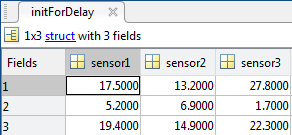11. In the Memory block dialog box, set Initial condition to `initForDelay`.

The Memory block output is an array of buses that requires initialization. Each signal element in the array of buses acquires an initial value from the corresponding field in the array of structures.

### Organize Parameters into Arrays of Structures

The base workspace contains many variables that the example model uses for block parameters. To reduce the number of workspace variables, package them into arrays of structures, and use the individual structure fields to specify block parameters.

A For Each Subsystem block can partition an array of values that you specify as a mask parameter. Each iteration of the subsystem uses a single partition of the array to specify block parameters. If you specify the parameter as an array of structures, each iteration of the subsystem can use one of the structures in the array.

1. Create an array of structures that parameterizes the For Each Subsystem block named `Burner_Analysis`, and store the array in the variable `paramsNormal`. Specify the structure fields by using the values of existing parameter variables, such as `gainNormal_1`, `offsetNormal_1`, and `initDelayed_1`.

```for i = 1:3 eval(['paramsNormal(' num2str(i) ').gain = gainNormal_' num2str(i) ';']) eval(['paramsNormal(' num2str(i) ').offset = offsetNormal_' num2str(i) ';']) eval(['paramsNormal(' num2str(i) ').init = initNormal_' num2str(i) ';']) end```

The variable contains an array of three structures that each has three fields: `gain`, `offset`, and `init`.

2. In the model, right-click the `Burner_Analysis` for-each subsystem and select Mask > Create Mask.

3. On the Parameters & Dialog pane of the dialog box, under Parameter, click Edit. For the new mask parameter, set Prompt to `Parameter structure` and Name to `paramStruct`. Click OK.

4. In the mask for the `Burner_Analysis` subsystem, set Parameter structure to `paramsNormal`.

5. Open the subsystem. In the For Each block dialog box, on the Parameter Partition pane, select the check box to partition the parameter `paramStruct`. Set Partition dimension to `2`.

6. For the blocks in the subsystem, set these parameters.

BlockParameter NameParameter Value
GainGain`paramStruct.gain`
Discrete-Time IntegratorInitial condition`paramStruct.init`
ConstantConstant value`paramStruct.offset`

7. Create an array of structures that parameterizes the `Burner_Analysis_Delay` for-each subsystem, and store the array in the variable `paramsForDelay`.

```for i = 1:3 eval(['paramsForDelay(' num2str(i) ').gain = gainDelayed_' num2str(i) ';']) eval(['paramsForDelay(' num2str(i) ').offset = offsetDelayed_' num2str(i) ';']) eval(['paramsForDelay(' num2str(i) ').init = initDelayed_' num2str(i) ';']) end```

8. At the top level of the model, right-click the `Burner_Analysis_Delay` for-each subsystem and select Mask > Create Mask.

9. On the Parameters & Dialog pane of the dialog box, under Parameter, click Edit. For the new mask parameter, set Prompt to `Parameter structure` and Name to `paramStruct`. Click OK.

10. In the mask for the For Each Subsystem block, set Parameter structure to `paramsForDelay`.

11. Open the subsystem. In the For Each block dialog box, on the Parameter Partition pane, select the check box to partition the parameter `paramStruct`. Set Partition dimension to `2`.

12. For the blocks in the subsystem, set these parameters.

BlockParameter NameParameter Value
GainGain`paramStruct.gain`
Discrete-Time IntegratorInitial condition`paramStruct.init`
ConstantConstant value`paramStruct.offset`

13. Clear the unnecessary variables from the base workspace.

```% Clear the old parameter variables that you replaced % with arrays of structures clear -regexp _ % Clear the iteration variables clear i```

The model requires few variables in the base workspace.

### Inspect Converted Model

To view the new signal and subsystem organization, update the diagram.The model input is an array of three buses. The model uses two for-each subsystems to execute the two algorithms on each of the three buses in the input array.

In the base workspace, arrays of structures replace the many variables that the model used. Mathematically, the modified model behaves the same way it did when you started because the arrays of structures contain the values of all the old variables.

Tip

You can log nonbus signals in a for-each subsystem. However, you cannot use signal logging for buses or arrays of buses from within a for-each subsystem. Either use a Bus Selector block to select the bus element signals that you want to log or add an Outport block outside of the subsystem and then log that signal. For details, see Log Signals in For Each Subsystems.

### Additional Examples of Working with For-Each Subsystems

#### Vectorize Algorithms Using For-Each Subsystems

This example shows how to simplify modeling of vectorized algorithms. Using For Each Subsystem blocks simplifies a model where three input signals are filtered by three identical Transfer Fcn blocks. This example also shows how to add more control to the filters by changing their coefficients for each iteration of the subsystem.

This model uses identical Transfer Fcn blocks to independently process each signal. A Vector Concatenate block concatenates the resulting output signals. This repetitive process is graphically complex and difficult to maintain. Adding another signal also requires significant reworking of the model.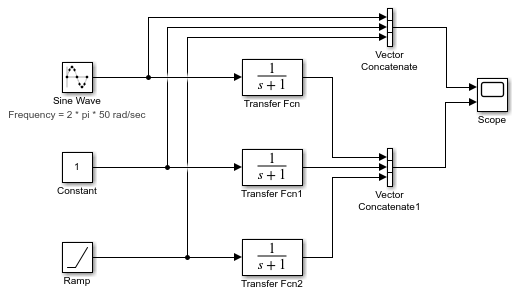You can simplify this model by replacing the repetitive operations with a single For Each Subsystem block.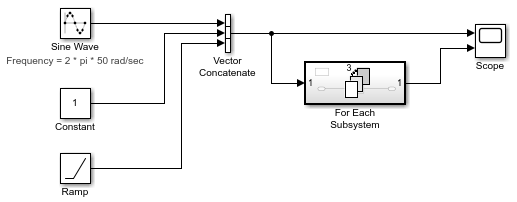The For Each Subsystem block contains a For Each block and a model representing the algorithm of the three blocks it replaces by way of the Transfer Fcn block. The For Each block specifies how to partition the input signal vector into individual elements and how to concatenate the processed signals to form the output signal vector. Every block that has a state maintains a separate set of states for each input element processed during a given execution step.For this example, the input signal is selected for partitioning. The Partition Dimension and Partition Width parameters on the For Each block are both set to `1` for the input.You can scale up this approach to add more signals without having to change the model significantly. This approach is easily scalable and graphically simpler.

Model Parameter Variation Without Changing Model Structure.  This example shows how to model parameter variation in an algorithm. It uses the for-each subsystem partitioning model from the previous example and creates different filters for each input signal while retaining model simplicity. An array of filter coefficients is fed to the For Each Subsystem block as a mask parameter marked for partitioning. In each iteration of the For Each Subsystem block, a partition of the filter coefficient array is fed to the Transfer Fcn block.

1. Open the model `ex_ForEachSubsystem_Partitioning`.

2. Create a mask for the For Each Subsystem block and add an editable mask parameter. Set the name to `FilterCoeffs` and the prompt to `Filter Coefficient Matrix`. For information on how to add a mask parameter, see Create a Simple Mask.3. Open the For Each Subsystem block. Inside the subsystem, open the For Each block dialog box.

4. In the Parameter Partition tab, select the check box next to the FilterCoeffs parameter to enable partitioning of this parameter. Keep the Partition Width and Partition Dimension parameters at their default value of 1.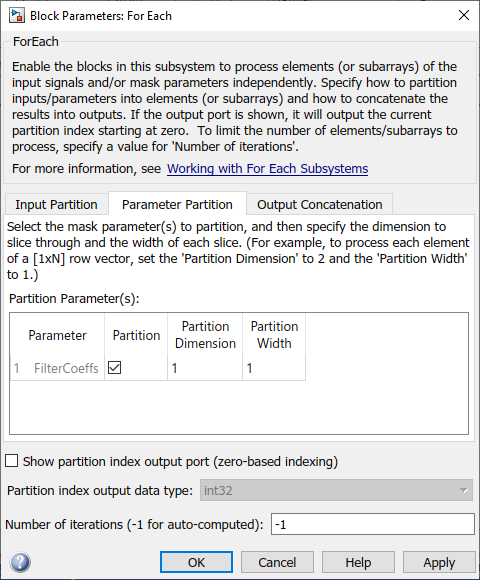5. Double-click the For Each Subsystem block and enter your filter coefficient matrix, having one row of filter coefficients for each input signal. For example, enter ```[0.0284 0.2370 0.4692 0.2370 0.0284; -0.0651 0 0.8698 0 -0.0651; 0.0284 -0.2370 0.4692 -0.2370 0.0284]``` to implement different fourth-order filters for each input signal.

6. In the For Each Subsystem block, double-click the Transfer Fcn block and enter `FilterCoeffs` for the Denominator Coefficients parameter. This setting causes the block to get its coefficients from the mask parameter.

The For Each Subsystem block slices the input parameter into horizontal partitions of width 1, which is equivalent to one row of coefficients. The parameter of coefficients starts as a single array.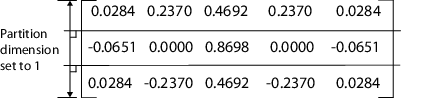It transforms from a single array into three rows of parameters.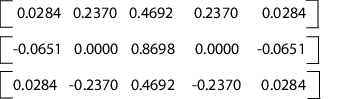#### Improved Code Reuse Using For-Each Subsystems

This example shows how you can design models to improve code reuse when you have two or more identical For Each Subsystem blocks. Consider the `ForEachReuse` model.The intent is for the three subsystems — `Vector SS1`, `Vector SS2`, and `Vector SS3` — to apply the same processing to each scalar element of the vector signal at their respective inputs. Because these three subsystems perform the same processing, it is desirable for them to produce a single shared Output (and Update) function for all three subsystems in the code generated for this model. For example, the `Vector SS3` subsystem contains these blocks.To generate a single shared function for the three subsystems, the configuration of the partitioning they perform on their input signals must be the same. For ```Vector SS1``` and `Vector SS3`, this configuration is straightforward because you can set the partition dimension and width to 1. However, in order for `Vector SS2` to also partition its input signal along dimension 1, insert a Math Function block to transpose the 1-by-8 row vector into an 8-by-1 column vector. To convert the output of the subsystem back to a 1-by-8 row vector using a second Math Function block set to the `transpose` operator. A reusable shared function can also be generated for the remaining two subsystems that process matrix signals. For more information about generating C code for the model, see Generate Reusable Code from For Each Subsystems (Simulink Coder).

### Limitations of For-Each Subsystems

The For Each Subsystem block has these limitations and workarounds.

LimitationWorkaround

You cannot log a bus or an array of buses directly in the for-each subsystem.

Use one of these approaches:

• Use a Bus Selector block to select the signals you want to log and mark those signals for signal logging.

• Attach the signal to an Outport block and log the signal outside the for-each subsystem.

You cannot log a signal inside a referenced model that is inside a for-each subsystem if either of these conditions exists:

• The for-each subsystem is in a model simulating in Rapid Accelerator mode.

• The for-each subsystem itself is in a model referenced by a Model block in Accelerator mode.

For the first condition, use Accelerator mode.

For the second condition, use Normal or Rapid Accelerator mode.

You cannot log the states of the blocks in a for-each subsystem.

Save and restore the simulation state.

You cannot use Normal mode to simulate a Model block inside a for-each subsystem.

Use Accelerator or Rapid Accelerator mode.

Reusable code is generated for two for-each subsystems with identical contents if their input and output signals are vectors (1-D or 2-D row or column vector). For n-D input and output signals, reusable code is generated only when the dimension along which the signal is partitioned is the highest dimension.

Permute the signal dimensions to transform the partition dimension and the concatenation dimension to the highest nonsingleton dimension for n-D signals.

The For Each Subsystem block does not support these features:

• You cannot include these blocks or S-functions inside a for-each subsystem:

• Data Store Memory, Data Store Read, or Data Store Write blocks

• The From Workspace block if the input data uses the `Structure with Time` format and has an empty time field

• To File blocks

• Goto and From blocks that cross the subsystem boundary

• Referenced model with simulation mode set to normal

• ERT S-functions

For a complete list of the blocks that support for-each subsystems, enter `showblockdatatypetable` in the MATLAB Command Window.

• You cannot use these types of signals:

• Signals with an external storage class inside the system

• Frame signals on subsystem input and output boundaries

• Variable-size signals of more than one dimension

• Creation of a linearization point inside the subsystem

• Propagating the Jacobian flag for the blocks inside the subsystem. You can check this condition in MATLAB using `J.Mi.BlockAnalyticFlags.jacobian`, where `J` is the Jacobian object. To verify the correctness of the Jacobian of the For Each Subsystem block:

• Look at the tag of the for-each subsystem Jacobian. If it is `“not_supported”`, then the Jacobian is incorrect.

• Move each block out of the for-each subsystem and calculate its Jacobian. If any block is `“not_supported”` or has a warning tag, the for-each subsystem Jacobian is incorrect.

• You cannot perform these types of code generation:

• Generation of a Simulink® Coder™ S-function target

• Simulink Coder code generation under both of these conditions:

• A Stateflow® or MATLAB Function block resides in the subsystem.

• This block tries to access global data outside the subsystem, such as Data Store Memory blocks or `Simulink.Signal` objects of `ExportedGlobal` storage class.

• PLC code generation

• In some cases, a model using a for-each subsystem may take longer to simulate in normal mode than it would take if the model were implemented using separate subsystems.